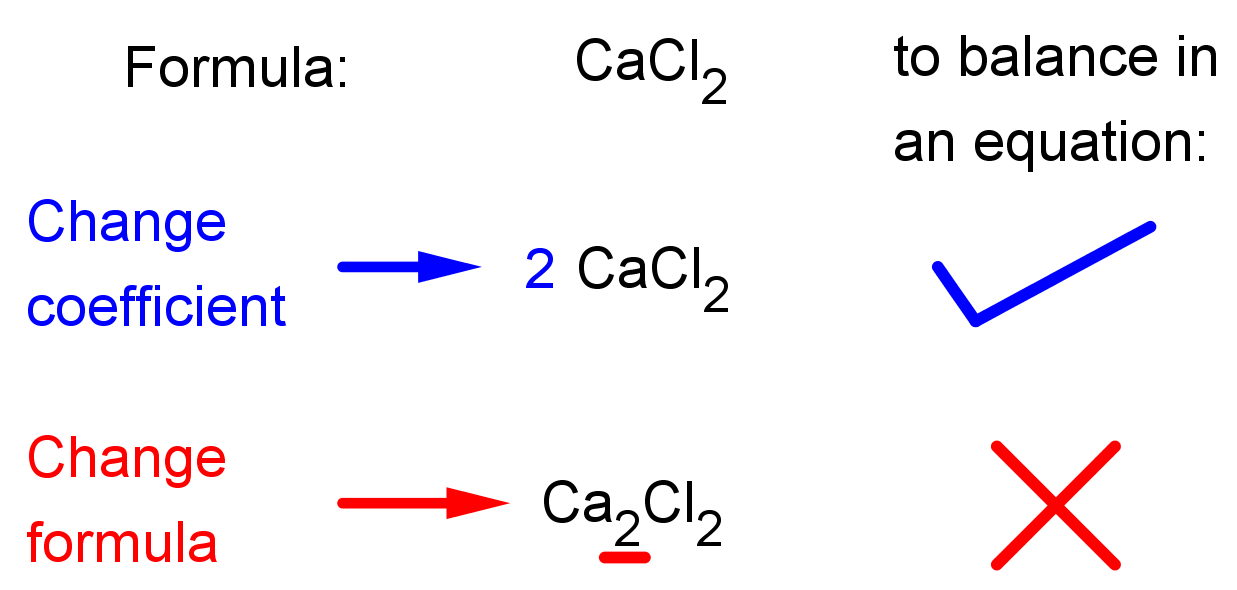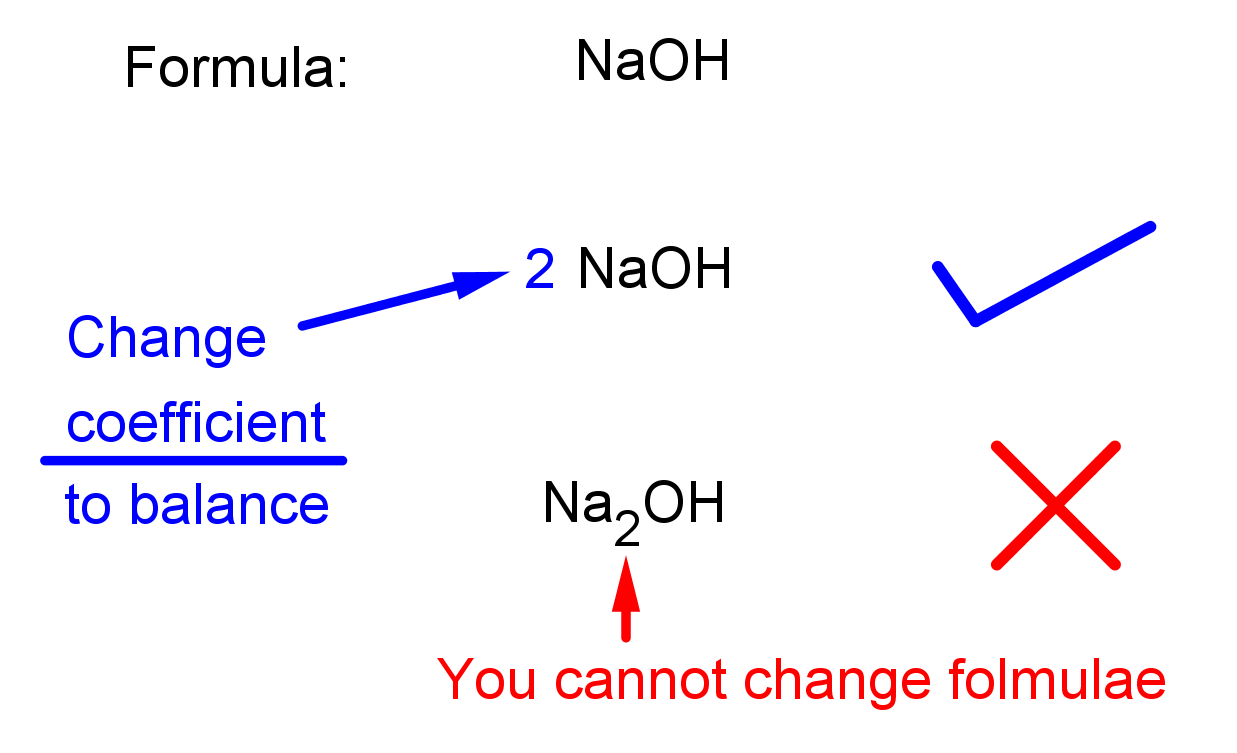# Balancing chemical equations

### Balancing chemical equations

#### Lessons

In this lesson, we will learn:
• To recall the conservation of mass and understand its meaning in chemical reactions.
• How to balance chemical equations including combustion and neutralization reactions.
• How to construct full chemical equations with known chemical formulae.

Notes:
• During chemical reactions, atoms are not created or destroyed, they are simply rearranged by breaking and forming bonds. This process is how chemical compounds are reacted to make different chemical compounds.

• This means the number of atoms of each element is exactly the same in the reactants and the products. You can use this information to check if an equation is balanced or not.

• The following rules need to be followed when balancing equations:
• Balancing step 1: Start with an element in the equation with only one input in the reactants and only one output in the products. Balance this first using coefficients.
• Balancing step 2: Once all these elements in step 1 are balanced, then balance the elements with more than one input or output.
• Balancing step 3: Count the number of atoms of each element in the products and the reactants. THEY MUST MATCH. If the number of atoms on both sides of the equations are not the same, your equation is not balanced.

• You CANNOT change the formula of a compound to balance an equation! You can only change the coefficient, the large number that goes in front of the chemical formula. See the illustrations below:
•• Introduction
Writing Chemical equations
a)
What is a chemical equation?

b)
What does the conservation of mass mean?

c)
A good method to balance equations quickly.

d)
A balancing mistake you need to avoid.

• 1.
Practice balancing equations.
Balance the equation:

H$_2$SO$_4 +$NaOH$\,$$\,$Na$_2$SO$_4 +$H$_2$O

• 2.
Balance the equation:

HCl$+$Mg(OH)$_2 \,$$\,$MgCl$_2 +$H$_2$O

• 3.
Balance the equation:

C$_3$H$_8 +$O$_2 \,$$\,$CO$_2 +$H$_2$O

• 4.
Balance the equation:

Na$_2$CO$_3 +$H$_3$PO$_4 \,$$\,$Na$_3$PO$_4 +$CO$_2 +$H$_2$O